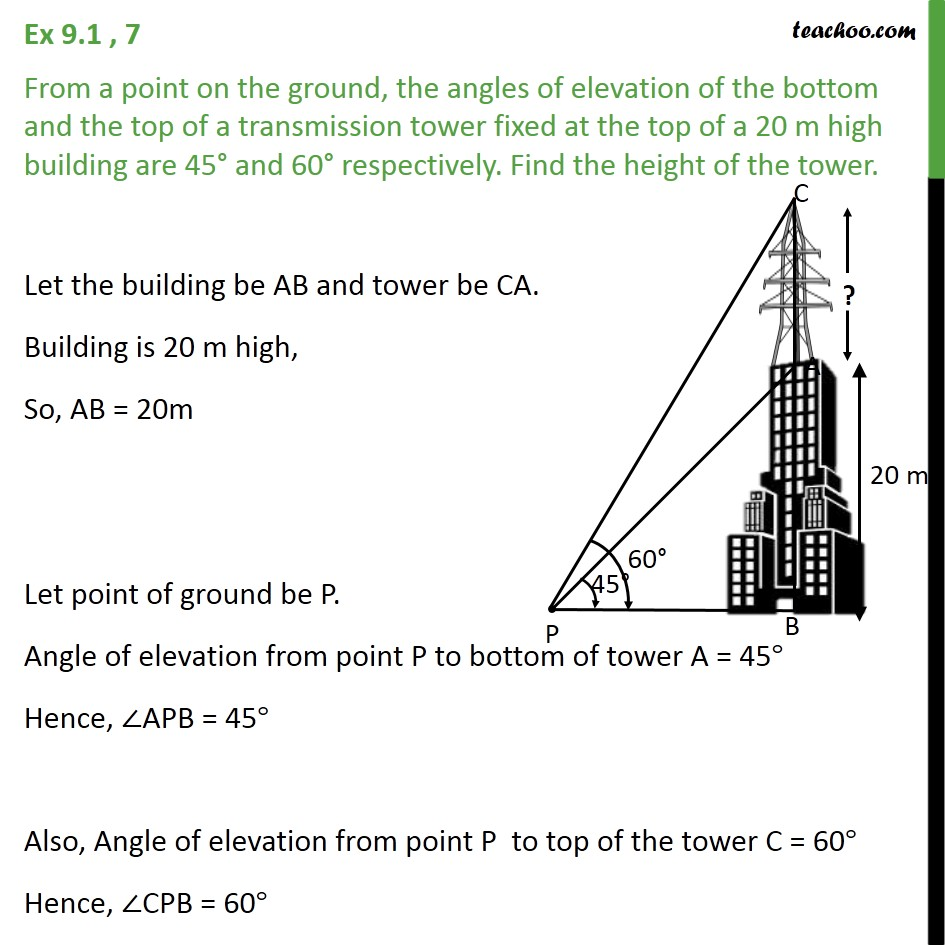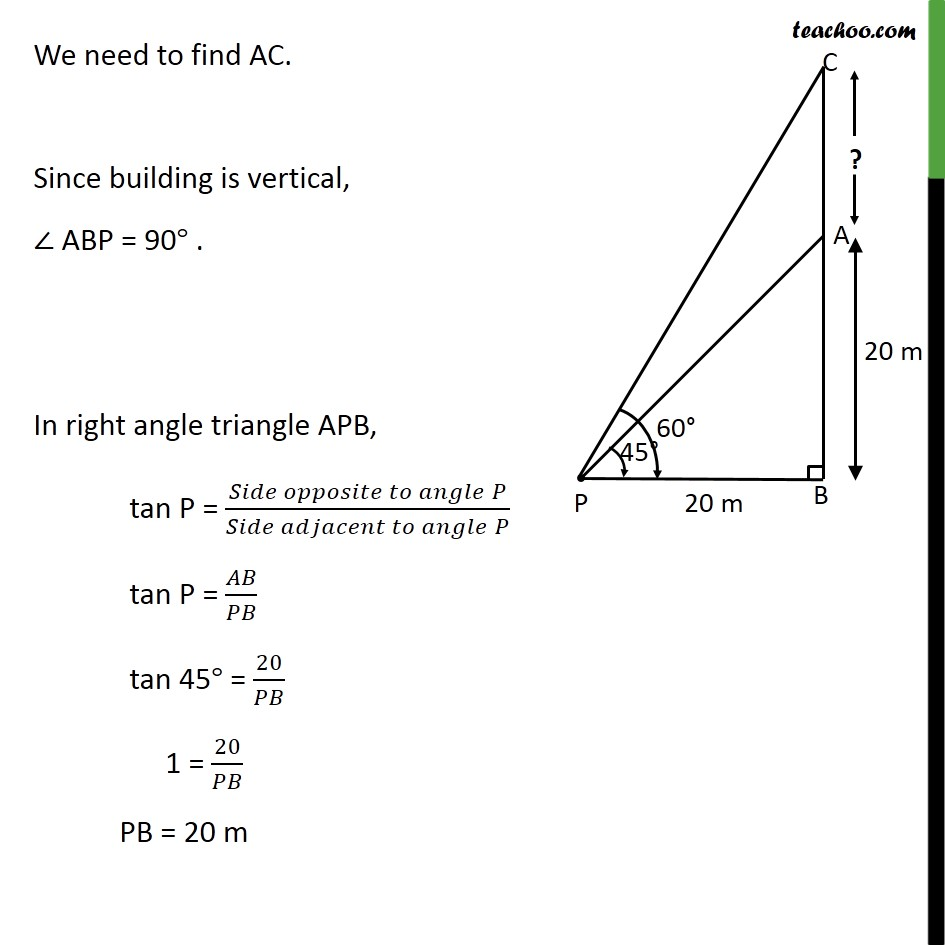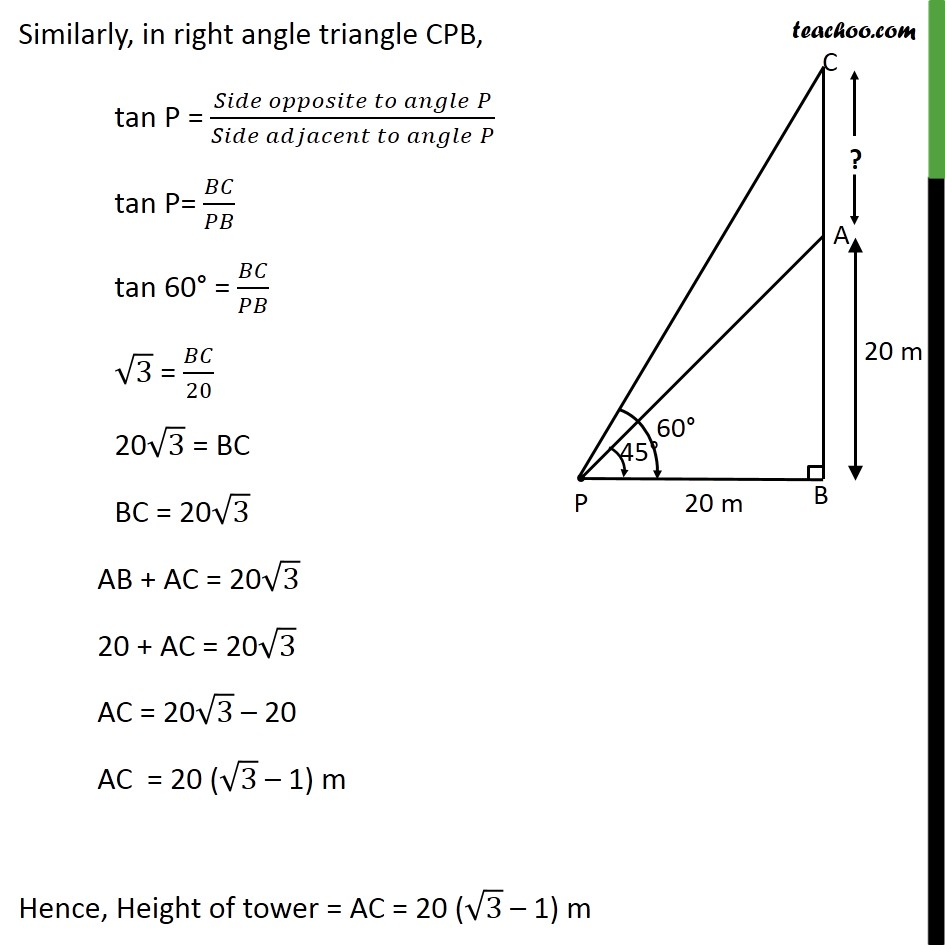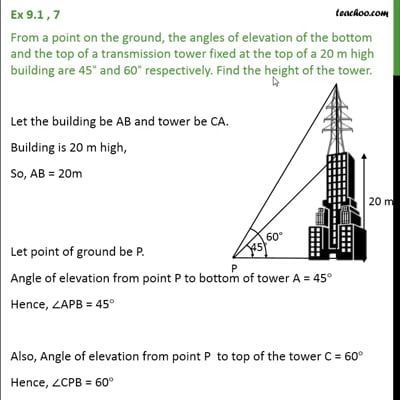Ex 9.1

Chapter 9 Class 10 Some Applications of Trigonometry
Serial order wiseThis video is only available for Teachoo black users

Solve all your doubts with Teachoo Black (new monthly pack available now!)

### Transcript

Ex 9.1 , 7 From a point on the ground, the angles of elevation of the bottom and the top of a transmission tower fixed at the top of a 20 m high building are 45 and 60 respectively. Find the height of the tower. Let the building be AB and tower be CA. Building is 20 m high, So, AB = 20m Let point of ground be P. Angle of elevation from point P to bottom of tower A = 45 Hence, APB = 45 Also, Angle of elevation from point P to top of the tower C = 60 Hence, CPB = 60 We need to find AC. Since building is vertical, ABP = 90 . In right angle triangle APB, tan P = ( " " )/( " " ) tan P = / tan 45 = 20/ 1 = 20/ PB = 20 m Similarly, in right angle triangle CPB, tan P = ( " " )/( " " ) tan P= / tan 60 = / 3 = /20 20 3 = BC BC = 20 3 AB + AC = 20 3 20 + AC = 20 3 AC = 20 3 20 AC = 20 ( 3 1) m Hence, Height of tower = AC = 20 ( 3 1) m